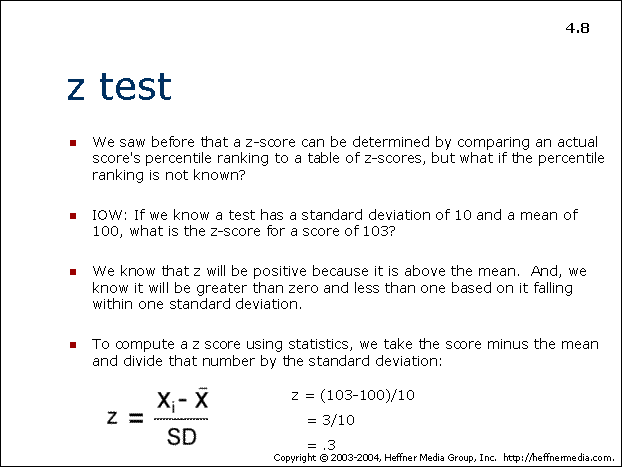# 08: Z-Test## z test

• We saw before that a z-score can be determined by comparing an actual score’s percentile ranking to a table of z-scores, but what if the percentile ranking is not known?
• IOW: If we know a test has a standard deviation of 10 and a mean of 100, what is the z-score for a score of a 103?
• We know that z will be positive because it is above the mean. And, we know it will be greater than zero and less than one based on it falling within one standard deviation.
• To compute a z score using statistics, we take the score minus the mean and divide that number by the standard deviation:

z = xi – x̄

SD

z = (103-100)/10

= 3/10

= .3